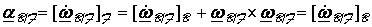Motion relative to moving frames

Two observers may provide different description of the same event if they are positioned in different frames. For example, a stationary point on the ground may look to an observer stationed on a merry-go-round as moving. Or, for example, passengers sitting in an airplane may consider each other as stationary, while an observer on earth may consider them to be moving.

Frames: To describe position in space we use a frame. The frame we use is frequently the background of the event we are observing, yet we can select any rigid body as our frame to reference our observations. The earth is the most common frame we use, but it is not the only one. For example, observations in the shuttle may be done relative to the “shuttle frame,” and then translated to earth frame.

Time derivatives and frames: The derivative of any variable with respect to time provides information on how it is changing with time. Since the same event when viewed from different frames may result in different observations, the time derivative of an event when taken relative to one frame may be different from the time derivative taken relative to another frame. For example, point A which is fixed to frame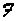has zero velocity as seen by an observer on frame, yet will have a downward velocity of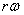if observed by an observer sitting at point O on the frame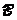, which is a disk rotating about O with angular velocityrelative to.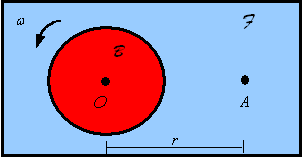One can relate the time derivative obtained from an observer in one frame to that obtained from an observer in another frame. Let the vector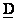be represent a quantity being observed by two observers, one on frameand one on frame. Let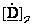denote the derivative ofobtained by the observer on, and let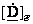denote the derivative ofobtained by the observer on.  These derivatives are related by following equation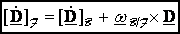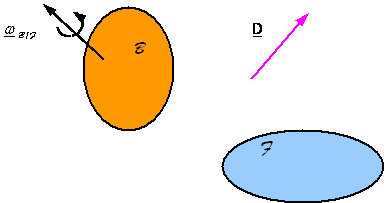where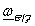is the angular velocity of framewith respect to frame.

Relating observations on velocity and acceleration: The position of a point P as it moves on its path can be described relative to the origin O of frameby the position vector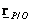or relative to the origin A of frameby the position vector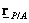. These vectors are related through the law of vector addition given by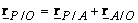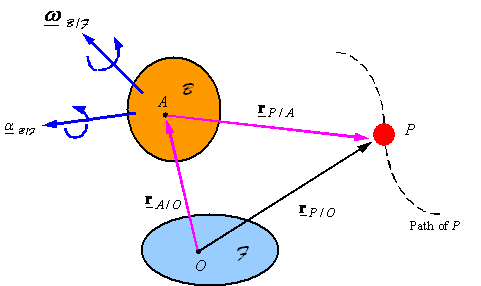Taking derivatives of both sides of the equation from the point of view of the observer inand application of the above relation to relate derivatives in two different frames results in the relation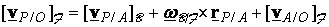The first term on the right hand side is the velocity as seen by an observer sitting at point A on frame, the second term on the right hand side is a modification of this velocity to account for the rotation of framewith respect to, and the third term on the right hand side is a modification of the first term to account for the translation ofwith respect to. The derivative of this gives the relation between the acceleration as observed from the two frames. After application of the rule for taking derivatives and some reorganization one gets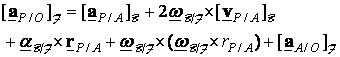The first term on the right hand side is the acceleration measured by an observer located at point A  on frame, the next three terms are modifications to correct for the rotation of framewith respect to, and the last term is to correct for the translation ofwith respect to.

Note: The derivative ofis the same in both frames. This follows from